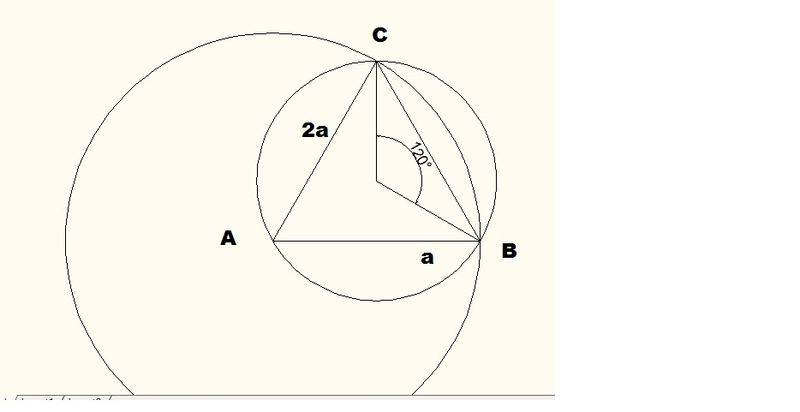# ABC is an equilateral triangle

## Homework Statement

Based on my understanding of the question the diagram should be like thisABC is an equilateral triangle with side 2a.Two circle are are drawn , with one of them crossing the points A,B and C.another circle crossess B and C with A as centre

Prove that the area enclosed by the two minor arcs BC are

(2a^2 / 9) x (3$\sqrt{}3$ - ∏)

## Homework Equations

Area of Segment of an Triangle
A= 1/2 a^2 (θ-sinθ)

## The Attempt at a Solution

Minor Arc Segment = Outer Segment - Inner Segment

Outer Segment

A=1/2 a^2 x (120 - sin120)
= (120-$\sqrt{}3$ / 2) a^2

Inner Segment

A=1/2 2a^2 x (60-sin60)
=(120-$\sqrt{}3$)a^2

Note:Update the working Steps in tmr ,sleep now! is 3am !

Last edited:

SteamKing
Staff Emeritus
Homework Helper

I think you are supposed to use radians to measure the angles.

HallsofIvy
Homework Helper

Yes. It doesn't matter for the "$sin(\theta)$" of course, but it does for the first $\theta$ in "$\theta- sin(\theta)$".

hmm thanks i think i got it

Suppose ?? Can I Continue to Asking In The Same Thread ?
By Using (Equation Reducible to Quadratic Form)

3-3cosX = 2sin^2 X
2sin^2X - 3cosX-3

As (sin^2 X) =1-cos2X / 2
2(1-cos2X/2) = 1-cos2X

1-1-cos2X -3cosX - 3
cos2X - 3cosX -3

As cos2X = 2 cos^2 X-1
So

2cos^2 X - 1 -3cosX - 3

Let cos X be y

2y^2 -1 - 3y - 3 = 0
2y^2 - 3y - 4 = 0
( ) ( ) = 0

Y1 = 2.35
Y2 = -0.85

No Real Number LOL!

Or Must Solving Using

Equation for the form a sin θ + - b cos θ = c
R cos A = θ--------------1
R sin A = θ --------------2